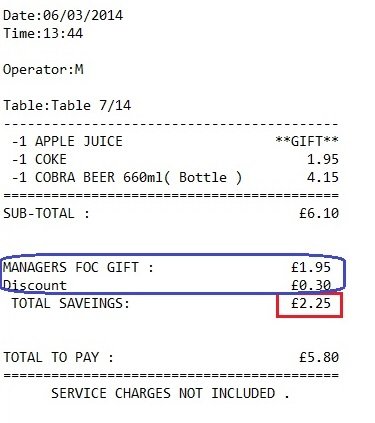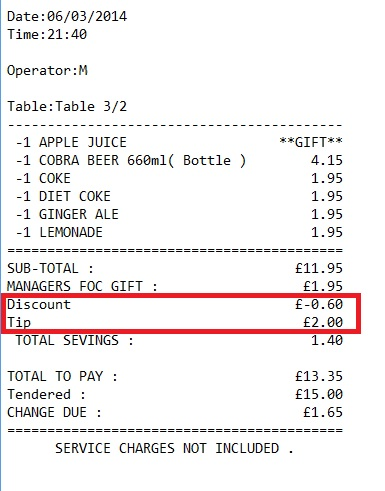# Calculating Total Saveings sum Rule (Total Gift + Discount)

Hi,

This may be simple but I need to know how to create the rule which calculates Total sum of discount provided.- Total value of Manager FOC GIFT + Total Discount.

The value would be printed under 'TOTAL SAVEINGS:1 Like

Can you post your print template?

Hi Emre,
I have attached the print template.

Below is an example of an transaction with GIFT, 5% Discount and £2 tip.

On the Print receipt TOTAL SAVINGS should be:£2.55 ( Gift+ Discount) and NOT £1.40

Currently if TIP and discount is included, the TOTAL SAVINGS is calculated as: Tip - Discount which is incorrect. the Issue is with following line.

TOTAL SEVINGS:|{DISCOUNT TOTAL}

Print ticket.zip (743 Bytes)You can enable “include tax” on tip calculation properties. Since tip is a fixed amount this won’t affect calculations and {DISCOUNT TOTAL} displays only discount amount.

So you need Discount + Gift value. You can calculate this with a custom expression.

``[=F(TN('{DISCOUNT TOTAL}') + TN('{ORDER STATE TOTAL:Gift}'))]``

I have enabled " include tax" on tip calculation and added the custom expression. I 'm now seeing following:

TIP figure NOT displayed on the Ticket
Total Savings figure and Total to Pay is calculated in correctly. As it minuses the Discount figure.

if I un-tick " Decrease Amount" from Discount calculation Properties then it adds the discount figure to ‘Total Savings’ figure and ‘Total to Pay’

Yes I’ve missed discount is a negative amount. You can just use a mathematical trick to calculate correct amount``````[=F(TN('{ORDER STATE TOTAL:Gift}')-TN('{DISCOUNT TOTAL}'))]
``````

You need to create a section for {SERVICES} for displaying tip amount. So I think your final template should be…

``````[LAYOUT]
-- General layout
<C10>
<L00>Date:{TICKET DATE}
<L00>Time:{TIME}
<L00>
<L00>Operator:{USER NAME}
<L00>
{ENTITIES}
<F>-
{ORDERS}
<F>=
[<J00>SUB-TOTAL:|£{PLAIN TOTAL}]

{DISCOUNTS}

<F>
<J10> TOTAL SEVINGS:|[=F(TN('{ORDER STATE TOTAL:Gift}')-TN('{DISCOUNT TOTAL}'))]
{TAXES}
{SERVICES}
<LF>
<DB>
<J10>TOTAL TO PAY:|£{TICKET TOTAL}
<J10>[Tendered:|£{TENDERED TOTAL}]
<J10>[CHANGE DUE:|£{CHANGE TOTAL}]
<EB>
<F>=
<C10>SERVICE CHARGES NOT INCLUDED.
<F>
<F>
<F>
<F>
<F>
[DISCOUNTS]
<J00>{CALCULATION NAME}|£{CALCULATION TOTAL}

[SERVICES]
<J00>{CALCULATION NAME}|£{CALCULATION TOTAL}

[TAXES]
<J00>{TAX NAME} %{TAX RATE}|{TAX AMOUNT}

[PAYMENTS]
<J00>{PAYMENT NAME}|{PAYMENT AMOUNT}

[ORDERS]
-- Default format for orders
<J00>- {QUANTITY} {NAME}|{TOTAL AMOUNT}
{ORDER TAGS}

{ORDER TAGS}

[ORDERS:Void]
-- Nothing will print for void lines

[ORDER TAGS]
-- Format for order tags
<J00> * {ORDER TAG NAME}|{ORDER TAG PRICE}

[ENTITIES:Table]
-- Table entity format
<L00>Table:{ENTITY NAME}/{TICKET TAGN:Number of Customers}

[ENTITIES:Deliverer]
-- Delivere entity format
<L00>Deliverer:{ENTITY NAME}
<L00>
[ENTITIES:Customer]
-- Customer entity format
<J00>Customer: {ENTITY NAME}|Tel: {ENTITY DATA:Phone}
Thank you Emra much appreciated!!!its working perfectly as it should now.